NCERT Exemplar Class 12 Chemistry Chapter 2 Solution are part of NCERT Exemplar Class 12 Chemistry. Here we have given NCERT Exemplar Class 12 Chemistry Chapter 2 Solution. https://www.cbselabs.com/ncert-exemplar-problems-class-12-chemistry-solution/

## NCERT Exemplar Class 12 Chemistry Chapter 2 Solution

Multiple Choice Questions

Single Correct Answer Type

Question 1. Which of the following unit is useful in relating concentration of solution with its vapour pressure?
(a) Mole fraction (b) Parts per million
(c) Mass percentage (d) Molality
Solution: (a) Mole fraction is useful in’relating vapour pressure with concentration of solution. According to Raoult’s law, the partial vapour pressure of each component in the solution is directly proportional to its mole fraction.
A is one component.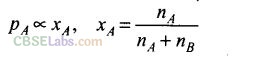NCERT exemplar class 12 chemistry chapter 2

Question 2. On dissolving sugar in water at room temperature solution feels cool to touch. Under which of the following case dissolution of sugar will be most rapid?
(a) Sugar crystals in cold water (b) Sugar crystals in hot water
(c) Powdered sugar in cold water (d) Powdered sugar in hot water
Solution: (d) Since the solution is cool to touch, the dissolution is endothermic. Therefore, high temperature will favour dissolution. Further, powdered sugar has large surface area and is favourable for dissolution.

Question 3. At equilibrium the rate of dissolution of a solid solute in a volatile liquid
solvent is
(a) less than the rate of crystallisation
(b) greater than the rate of crystallisation
(c) equal to the rate of crystallisation
(d) zero
Solution: (c) At equilibrium the rate of dissolution of solid solute is equal to rate of crystallisation.

NCERT exemplar class 12 chemistry

Question 4. A beaker contains a solution of substance ‘A’. Precipitation of substance ‘A’ takes place when small amount of ‘A’ is added to the solution. The solution is
(a) saturated (b) supersaturated
(c) unsaturated (d) concentrated
Solution: (b) When small amount of solute is added to its solution and it does not dissolve and get precipitated then this solution is supersaturated solution.

Question 5. Maximum amount of a solid solute that can be dissolved in a specified
amount of a given liquid solvent does not depend upon
(a) temperature (b) nature of solute
(c) pressure (d) nature of solvent
Solution: (c) Solubility of a solid in liquid does not depend on pressure because solid is practically incompressible.

number of moles of the mixture is the sum of the number of moles of each components that existed in the mixture, the mole fraction equation becomes.

NCERT exemplar class 12 chemistry solutions

Question 6. Low concentration of oxygen in the blood and tissues of people living at high altitude is due to
(a) low temperature
(b) low atmospheric pressure
(c) high atmospheric pressure
(d) both low temperature and high atmospheric pressure
Solution: (b) At high altitudes the atmospheric pressure is less but the body temperature remains same hence concentration of oxygen in the air as well as blood is less.

Question 7. Considering the formation, breaking and strength of hydrogen bond, predict which of the following mixture will show a positive deviation from Raoulf s law?
(a) Methanol and acetone (b) Chloroform and acetone
(c) Nitric acid and water (d) Phenol and aniline
Solution: (a) Mixture of Methanol and acetone shows positive deviation because methanol-methanol and acetone-acetone interactions are more than methanol-acetone. The more number of hydrogen bonds are broken the less number of new H-bonds are formed.

NCERT exemplar chemistry class 12

Question 8. Colligative properties depend on
(a) the nature of the solute particles dissolved in solution
(b) the number of solute particles in solution
(c) the physical properties of the solute particles dissolved in solution
(d) the nature of solvent particles
Solution: (b) Colligative properties depend upon the number of solute particles in the solution and independent of its nature.

Question 9. Which of the following aqueous solution should have the highest boiling point?
(a) l.OMNaOH (b) 1.0MNa2SO4
(c) 1.0MNH4NO3 (d) l.OMKNO3
Solution: (b) 1.0 M Na2SO4 since it furnishes maximum number of ions (2Na+ + SO4).

class 12 chemistry exemplar solutions

Question 10. The unit of ebullioscopic constant is , – ;Solution: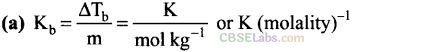The unit of ebullioscopic constant is K kg mol-1 or K molality-1.

Question 11. In comparison to a 0.01 M solution of glucose, the depression in freezing point of a 0.01 M MgCl2 solution is 1
(a) the same (b) about twice
(c) about three times (d) about six times
Solution: (c) 0.01 M solution of glucose does not ionize while 0.01 M MgCl2 solution furnishes 3 ions (Mg2+ + 2Cl) in the solution, hence the value of colligative property for MgCl2 solution is about 3 times.

Osmotic Pressure Calculator analyzes the amount of pressure required to completely halt the osmosis.

Question 12. An unripe mango placed in a concentrated salt solution to prepare pickle, shrivels because
(a) it gains water due to osmosis
(b) it loses water due to reverse osmosis
(c) it gains water due to reverse osmosis
(d) it loses water due to osmosis
Solution: (d) Water starts moving out of mango (lower concentration) to the salt solution (higher concentration) due to osmosis.

Question 13. At a given temperature, osmotic pressure of a concentrated solution of a substance
(a) is higher than that of a dilute solution
(b) is lower than that of a dilute solution
(c) is same as that of a dilute solution
(d) cannot be compared with osmotic pressure of dilute solution
Solution: (a)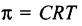where C is concentration of the solution. So, the higher the concentration of solution at given temperature the higher will be the osmotic pressure.

Question 14. Which of the following statement is false?
(a) Two different solutions of sucrose of same molality-prepared in different solvents will have the same depression in freezing point.
(b) The osmotic pressure of a solution is given by the equation 3.14(pi) = CRT (where C is the molarity of the solution).
(c) Decreasing order of osmotic pressure for 0.01 M aqueous solutions of barium chloride, potassium chloride, acetic acid and sucrose is
BaCl2> KCl > CH2COOH > sucrose
(d) According to Raoult’s law, the vapour pressure exerted by a volatile component of a solution is directly proportional to its mole fraction in the solution.
Solution: (a) Value of A is different for different solvents. Thus, according to relation,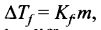even for same molal solutions depression in freezing point will be different.

Question 15. The value of van’t Hoff factor.s for KCl, NaCl and K2SO4, respectively, are
(a) 2, 2 and 2 (b) 2, 2 and 3 (c) 1, 1, and 2 (d) 1, 1 and 1
Solution: (b) KCl (K+ + Cl ) and NaCl (Na+ + Cl ) ionize to give 2 ions and K2SO4(2K+ + SO4) ionizes to give 3 ions thus, van’t Hoff factors for KCl, NaCl and K2SO4 are 2, 2 and 3 respectively.

Question 16. Which of the following statement is false?
(a) Units of atmospheric pressure and osmotic pressure are the same.
(b) In reverse osmosis, solvent molecules move through a semipermeable membrane from a region of lower concentration of solute to a region of higher concentration.
(c) The value of molal depression constant depends on nature of solvent.
(d) Relative lowering of vapour pressure, is a dimensionless quantity.
Solution: (b) In reverse osmosis, solvent molecules move through a semipermeable
membrane from higher concentration of solute to lower concentration.

Question 17. Value of Henry’s constant KH
(a) increases with increase in temperature
(b) decreases with increase in temperature
(c) remains constant
(d) first increases then decreases
Solution: (a) Value of Henry’s constant increases with increase in temperature.

Question 18. The value of Henry’s constant KH is
(a) greater for gases with higher solubility
(b) greater for gases with lower solubility
(c) constant for all gases
(d) not related to the solubility of gases
Solution: (b) The higher the value of KH, the lower is the solubility of gas in the liquid.

Question 19. Consider the figure and mark the correct option.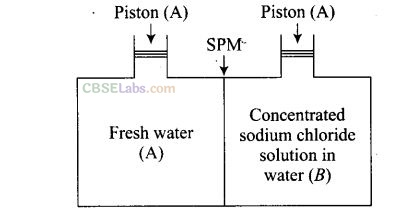(a) Water will move from side (A) to side (B) if a pressure lower than osmotic pressure is applied on piston (B).
(b) Water will move from side (B) to side (A) if a pressure greater than osmotic pressure is applied on piston (B).
(c) Water will move from side (B) to side (A) if a pressure equal to osmotic pressure is applied on piston (B).
(d) Water will move from side (A) to side (B) if pressure equal to osmotic pressure is applied on piston (A).
Solution: (b) Water will move from side 3 (concentrated sodium chloride solution) to side A (fresh water) if a pressure greater than osmotic pressure is applied on piston B.

Question 20. We have three aqueous solutions of NaCl labelled as ‘A’, ‘B’ and ‘C’ with
concentrations 0.1 M, 0.01 M and 0.001 M, respectively. The value of van’t Hoff factor for these solutions will be in the order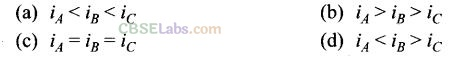Solution: (c) The value of van’t Hoff’s factor will be iA=iB =iC due to complete dissociation of strong electrolyte (NaCl) in dilute solutions. On complete dissociation value of i for NaCl is 2.

Question 21. On the basis of information given below mark the correct option. Information:
(I) In bromoethane and chloroethane mixture intermolecular interactions of A-A and B-B type are nearly same as A-B type interactions.
(II) In ethanol and acetone mixture A-A or B-B type intermolecular interactions are stronger than A-B type interactions.
(III) In chloroform and acetone mixture A-A or B-B type intermolecular interactions are weaker than A-B type interactions.
(a) Solution (II) and (III) will follow Raoult’s law.
(b) Solution (I) will follow Raoult’s law.
(c) Solution (II) will show negative deviation from Raoult’s law.
(d) Solution (III) will show positive deviation from Raoult’s law.
Solution: (b) Solution A will follow Raoult’s law due to nearly same interactions between A-A, B-B and A-B. The solution formed will be nearly ideal.

Question 22. Two beakers of capacity 500 mL were taken. One of these beakers, labelled “A”, was filled with 400 mL water whereas’the beaker labelled “B” was filled with 400 mL of 2 M solution of NaCl. At the same temperature both the beakers were placed in closed containers of same material and same capacity as shown in figure.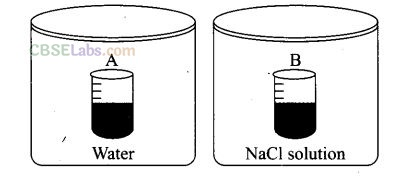At a given temperature, which of the following statement is correct about the vapour pressure of pure water and that of NaCl solution.
(a) Vapour pressure in container (A) is more than that in container (B).
(b) Vapour pressure in container (A) is less than that in container (B).
(c) Vapour pressure is equal in both the containers.
(d) Vapour pressure in container (B) is twice the vapour pressure in container (A).
Solution: (a) Since NaCl is a non-volatile solute, it will reduce vapour pressure of the solution. Hence, vapour pressure of pure water in container A is more than in container B.

Question 23. If two liquids A and B form minimum boiling azeotrope at some specific composiiion’hien
(a) A-B interactions are stronger than those between A-A or B-B.
(b) vapour pressure of solution increases because more number of molecules of liquids A and B can escape from the solution.
(c) vapour pressure of solution decreases because less number of molecules of only one of the liquids escape from the solution.
(d) A-B interactions are weaker than those between A-A or B-B.
Solution: (d) If A-B interactions < A-A or B-B the vapour pressure will be more and the result will be positive deviation. The solutions which show positive deviation form minimum boiling azeotropes.

Question 24. 4 L of 0.02 M aqueous solution of NaCl was diluted by adding one litre of water. The molality of the resultant solution is
(a) 0.004 (b) 0.008 (c) 0.012 (d) 0.016
Solution: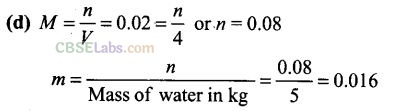Question 25. On the basis of information given below, mark the correct option. Information: On adding acetone to methanol some of the hydrogen bonds between methanol molecules break.
(a) At specific composition methanol-acetone mixture will form minimum boiling azeotrope and will show positive deviation from Raoulf s law.
(b) At specific composition methanol-acetone mixture forms maximum boiling azeotrope and will show positive deviation from Raoulf s law.
(c) At specific composition methanol-acetone mixture will form minimum boiling azeotrope and will show-negative deviation from Raoult’s law.
(d) At specific composition methanol-acetone mixture will form maximum boiling azeotrope and will show negative deviation from Raoulf s law.
Solution: (a) At specific composition methanol-acetone mixture will show positive
deviation from Raoulf s law as it has lesser interactions than methanol- methanol and acetone-acetone interactions. Hence it forms minimum boiling azeotrope.

Question 26. KH value for Ar(g),CO2(g), HCHO(g) and CH4(g) are 40.39, 1.67, 1.83 x 10.5
and 0.413 respectively.
Arrange these gases in the order of their increasing solubility.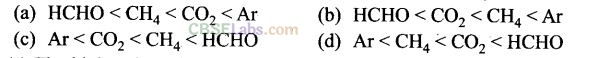Solution: (c) The higher the value of KH, the lower is the solubility of the gas in the liquid. Hence the order of increasing solubility of the gases will be Ar <  CO2<CH4< HCHO

Question 27. Which of the following factor(s) affect the solubility of a gaseous solute in the fixed volume of liquid solvent?
(i) Nature of solute (ii) Temperature
(iii) Pressure
(a) (i) and (iii) at’constant T (b) (i) and (ii) at constant P
(c) (ii) and (iii) only (d) (iii) only
Solution: (a, b) At constant temperature, the solubility of a gaseous solute in liquid depends on nature of solute and pressure. At constant pressure, solubility is dependent upon nature of solute and temperature.

Question 28. Intermolecular forces between two benzene molecules are nearly of same strength as those between two toluene molecules. For a mixture of benzene and toluene, which of the following are not true?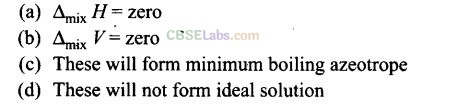Solution: (c, d) For an ideal solution,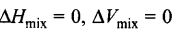and benzene and toluene will form an ideal solution. Also, ideal solutions do not form minimum boiling azeotropes.

Question 29. Relative lowering of vapour pressure is a colligative property because
(a) it depends on the concentration of a non-electrolyte solute in solution and does not depend on the nature of the solute molecules.
(b) it depends on number of particles of electrolyte solute in solution and does not depend on the nature of the solute particles.
(c) it depends on the concentration of a non-electrolyte solute in solution as well as on the nature of the solute molecules.
(d) it depends on the concentration of an electrolyte or a non-electrolyte solute in solution as well as on the nature of solute molecules
Solution:(a, b) Relative lowering of vapour pressure depends on concentration of non¬electrolyte solute in solution and number of particles of electrolyte solute. It does not depend on nature of solute in both conditions.

Question 30.van’t Hoff factor i is given by the expressionSolution: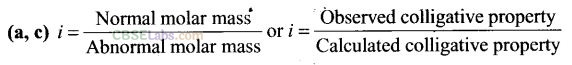Question 31. Isotonic solutions must have the same
(a) solute (b) density
(c) elevation in boiling point (d) depression in freezing point
Solution: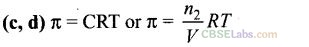Isotonic solutions must have same osmotic pressure at a given temperature hence must have same volume and number of moles i.e., same molar concentration. Thus, the isotonic solutions have same elevation in boiling point, and depression in freezing point.

Question 32. Which of the following binary mixtures will have same composition in liquid and vapour phase?
(a) Benzene-Toluene (b) Water-Nitric acid
(c) Water-Ethanol (d) 6-Hexane-n-Heptane
Solution: (b, c) Non-ideal solution mixtures with same composition in liquid and vapour phase are azeotropes. Water-nitric acid has maximum boiling azeotrope and water-ethanol has a minimum boiling azeotrope.

Question 33. In isotonic solutions
(a) solute and solvent both are same
(b) osmotic pressure is same
(c) solute and solvent may or may not be same
(d) solute is always same solvent may be different
Solution: (b, c) For isotonic solutions osmotic pressure is same, solute or solvent may not be same.

Question 34. For a binary ideal liquid solution, the variation in total vapour pressure versus composition of solution it given by which of the curves?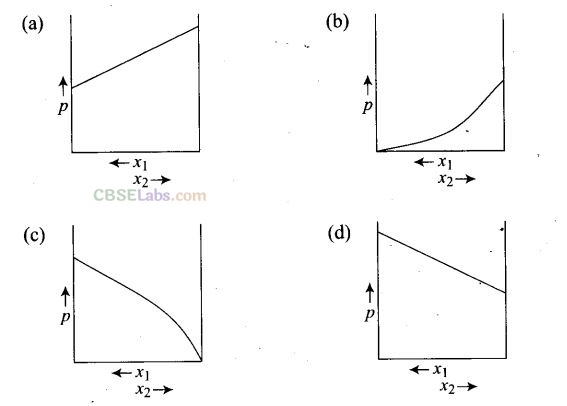Solution: (a,d)for idea solution.Question 35. Colligative properties are observed when
(a) a non-volatile solid is dissolved in a volatile liquid
(b) a non-volatile liquid is dissolved in an another volatile liquid
(c) a gas is dissolved in a non-volatile liquid
(d) a volatile liquid is dissolved in an another volatile liquid
Solution: (a, b) Colligative properties are observed when a non-volatile solid or liquid are dissolved in a volatile liquid.

Short Answer Type Questions

Question 36. Components of a binary mixture of two liquids A and B were being separated by distillation. After some time separation of components stopped and composition of vapour phase became same as that of liquid phase. Both the components started coming in the distillate. Explain why does this happen?
Solution: Since both the components are coming in the distillate and composition of liquid and vapour phase become same, this shows that liquids have formed azeotropic mixture. Therefore, these components cannot be separated at this stage by distillation.

Question 37. Explain why on addition of 1 mol of NaCl to 1 litre of water, the boiling point of water increases, while addition of 1 mol of methyl alcohol to one litre of water decreases its boiling point.
Solution: NaCl is a non-volatile solute, therefore, addition of NaCl to water lowers the vapour pressure of water. As a result boiling point of water increases. On the other hand, methyl alcohol is more volatile than water, therefore its addition increases the total vapour pressure over the solution. As a result, boiling point of water decreases.

Question 38. Explain the solubility rule “like dissolves like” in terms of intermolecular forces that exist in solutions.
Solution: A substance dissolves in a solvent if the intermolecular interactions are similar in both the components. For example, polar solutes dissolve in polar solvents and non-polar solutes in non-polar solvents. Thus, we can say “like dissolves like”.

Question 39. Concentration terms such as mass percentage, ppm, mole fraction and molality are independent of temperature, however molarity is a function of temperature. Explain.
Solution: Molarity of a solution is defined as the number of moles of solute dissolved per litre of solution. Since volume depends on temperature and changes with change in temperature, therefore, the molarity will also change with change in temperature. On the other hand, mass does not change with change in temperature, and therefore, concentration terms such as mass percentage, mole fraction and molality which do not involve volume are independent of temperature.

Question 40. What is the significance of Henry’s law constant KH?
Solution: According to Henry’s law:Question 41. Why are aquatic species more comfortable in cold water in comparison to warm water?
Solution: At a given pressure the solubility of oxygen in water increases with decrease in temperature. Therefore, the concentration of oxygen in sea is more in cold water and thus presence of more oxygen at lower temperature makes the aquatic species more comfortable in cold water.

Question 42. (a) Explain the following phenomena with the help of Henry’s law.
(i) Painful condition known as bends.
(ii) Feeling of weakness and discomfort in breathing at high altitude.
(b) Why does soda water bottle kept at room temperature fizz on opening?
Solution: (a)
(i) Deep sea divers depend upon compressed air for breathing at high pressure under water. The compressed air contains  N2 in addition to 02, which are not very soluble in blood at normal pressure. However, at great depths when the diver breathes in compressed air from the supply tank, more N2 dissolves in the blood and other body fluids because the pressure at that depth is far greater than the surface atmospheric pressure. When the diver comes towards the surface, the pressure decreases, N2 comes out of the body quickly forming bubbles in the blood stream. These bubbles restrict blood flow, affect the transmission of nerve impulses. The bubbles can even burst the capillaries or block them and starve the tissues of 02. This condition is called the bends, which are painful and life-threatening.
(ii) At high altitudes the partial pressure of 02 is less than that at the ground level. This results in low concentration of oxygen in the blood and tissues of the people living at high altitudes or climbers. The low blood oxygen causes climbers to become weak and unable to think clearly known as anoxia.
(b) To increase the solubility of C02 in soft drinks, the soda water bottles are sealed under high pressure. When the bottle is opened at room temperature under normal atmosphere conditions, the pressure inside the bottle decreases to atmospheric pressure and excess C02 fizzes out.

Question 43. Why is the vapour pressure of an aqueous solution of glucose lower than that of water?
Solution: In pure liquid water, the entire surface of liquid is occupied by the molecules of water. When a non-volatile solute, such as glucose is dissolved in water some of the surface is covered by non-volatile glucose molecules. Therefore, the fraction of surface covered by the solvent molecules escaping. As a result
number of solvent molecules escaping from the surface also gets reduced and consequently the vapour pressure of aqueous solution of glucose is reduced.

Question 44. How does sprinkling of salt help in clearing the snow covered roads in hilly areas? Explain the phenomenon involved in the process.
Solution: When salt is spread over snow covered roads, it lowers the freezing point of water to such an extent that water does not freeze to form ice. As a result, the snow starts melting from the surface and therefore, it helps in clearing the roads. Hence, common salt acts as de-icing agent.

Question 45. What is a “semipermeable membrane”?
Solution: A membrane that permits the flow of solvent molecules not the solute molecules is called semipermeable membrane. During osmosis and reverse osmosis, only solvent molecules move across the semipermeable membrane.

Question 46. Give an example of a material used for making semipermeable membrane for carrying out reverse osmosis.
Solution: Cellulose acetate, potassium ferrocyanide, etc. are used as semipermeable membrane for carrying out reverse osmosis.

Matching Column Type Questions

Question 47. Match the items given in Column I and Column II.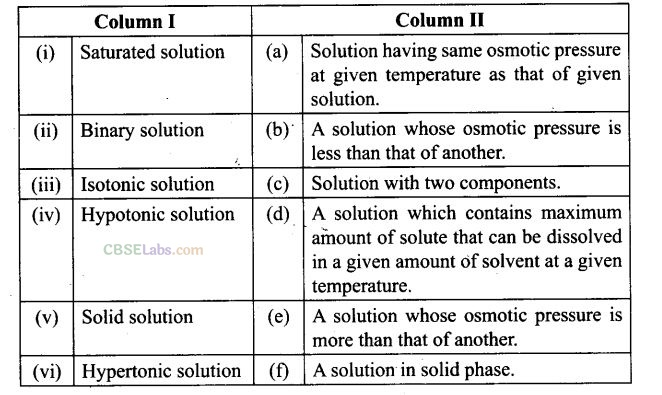Solution: (i —> d), (ii —> c); (iii—> a); (iv b), (v —> f); (vi —> e)
(i) Saturated solution: A solution which contains maximum amounts of solute that can be dissolved in a given amounts of solute that can be dissolved in a given amount of solvent at a given temperature.
(ii) Binary solution: A solution with two components is known as binary solution.
(iii) Isotonic solution: A solution having same osmotic pressure at a given temperature as that of given solution is known as isotonic solution.
(iv) Hypotonic solution: A solution whose osmotic pressure is less than another is known as hypotonic solution.
(v) Solid solution: A solution in solid phase is known as solid solution.
(vi) Hypertonic solution: A solution whose osmotic pressure is greater than that of another is known as hypertonic solution.

Question 48. Match the items given in Column 1 with the type of solutions given in Column II.Solution: (i -» e), (ii -» c), (iii -» d); (iv —> b), (v —> a)
(i) Soda water: A solution of gas in liquid, e.g.,CO2  in soft drinks.
(ii) Sugar solution: A solution of solid in liquid in which sugar particles (solid) are dissolved in water (liquid).
(iii) German silver: This is an alloy which is a solid solution of solid in solid. It is an alloy of Cu, Zn and Ni.
(iv) Air: A solution of gas in gas..Air is a mixture of various gases.
(v) Hydrogen gas in palladium: This is an example of solution of gas in solid. This is used as a reducing agent.

Question 49. Match the laws given in Column I with expressions given in Column II.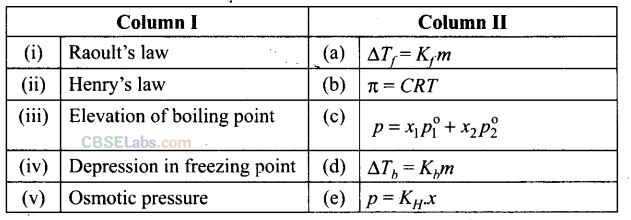Solution: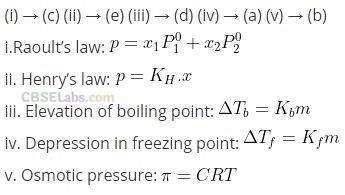Question 50. Match the terms given in Column I with expression given in Column II.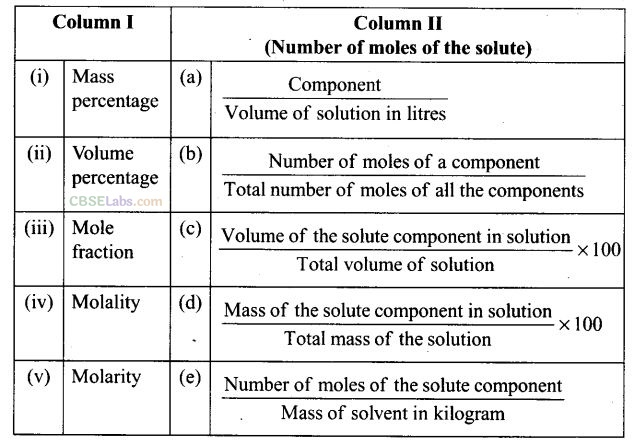Solution. (i —» d), (ii —» c), (iii —» b), (iv -> e), (v ->a)

Assertion and Reason Type Questions

In the following questions, a statement of Assertion (A) followed by a statement of
Reason (R) is given. Choose the correct answer out of the following choices:
(a) Assertion and reason both are correct statements and reason is the correct explanation for Assertion.
(b) Assertion and reason both are correct statements but reason is not the correct explanation for Assertion.
(c) Assertion is correct statement but reason is wrong.
(d) Assertion and reason both are incorrect.
(e) Assertion is wrong but reason is correct.

Question 51. Assertion (A): Molarity of a solution in liquid state changes with temperature. Reason (R): The volume of a solution changes with change in temperature.
Solution: (a) Molarity changes with temperature because volume changes with temperature.

Question 52. Assertion (A): When methyl alcohol is added to water, boiling point of water increases. Reason (R): When a volatile solute is added to a volatile solvent, elevation in boiling point is observed.
Solution: (d) When methyl alcohol (volatile) is added to water, boiling point of water decreases because vapour pressure increases when volatile solute is added to volatile solvent;

Question 53. Assertion (A): When NaCl is added to water, a depression in freezing point is observed. Reason (R): The lowering of vapour pressure of a solution causes depression in the freezing point.
Solution: (a) When a non-volatile solute is added to water, freezing point lowers due to lowering of vapour pressure.

Question 54. Assertion (A): When a solution is separated from the pure solvent by a semipermeable membrane, the solvent by a semipermeable membrahe, the solvent molecules pass through it from pure solvent side to the solution side. Reason (R): Diffusion of solvent occurs from a region of high concentration solution to a region of low concentration solution.
Solution: (c) Solvent molecules pass through the semipermeable membrane from region of low concentration solution to the region of high concentration solution.

Long Answer Type Questions

Question 55. Define the following modes of expressing the concentration of a solution. Which of these modes are independent of temperature and why?
(i) w/w (mass percentage)
(ii) x (mole fraction)
(iii) VIV (volume percentage)
(iv) M (molarity)
(v) wIV (mass by volume percentage)
(vi) m (molality)
(vii) ppm (parts per million)
Solution: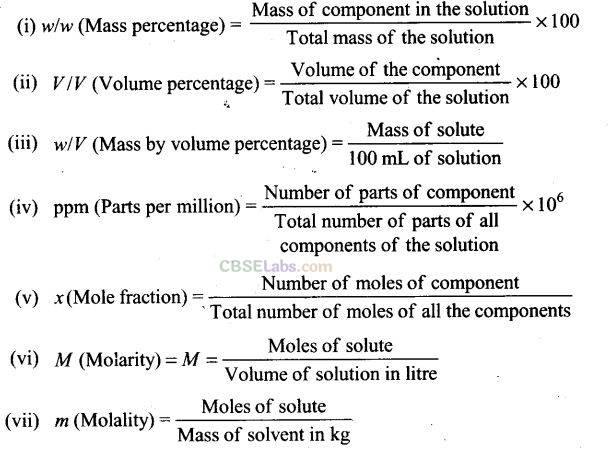Mass percentage, ppm, mole fraction and molality are independent of temperature since mass does not change with temperature.

Question 56. Using Raoult’s law explain how the total vapour pressure over the solution is related to mole traction of components in the given solutions.
(a) CHCl2 andCH4Cl2(l)
(b) NaCl(s) and H2O(l)
Solution:Question 57. Explain the terms ideal and non-ideal solutions in the light of forces of interactions operating between molecules in liquid solutions.
Solution: Ideal solutions: The solutions which obey Raoult’s law over the entire range of concentration are known as ideal solution. For an ideal solution,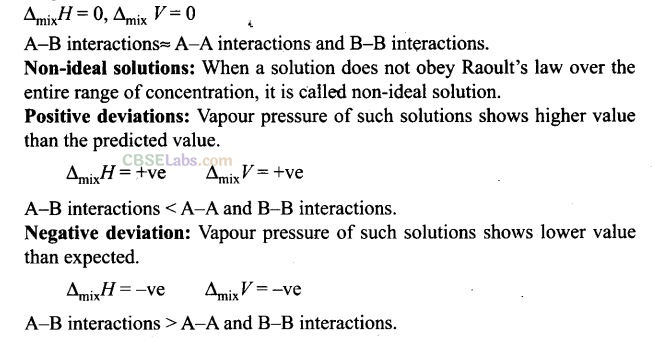Question 58. Why is it not possible to obtain pure ethanol by fractional distillation? What general name is given to binary mixtures which show deviation from Raoult’s law and whose components cannot be separated by fractional distillation? How many types of such mixtures are there?
Solution: The solution or mixture having same composition in liquid as well as in vapour phase and boils at a constant temperature is known as azeotropes. Due to constant composition it cannot be separated by fractional distillation. There are two types of azeotropes
(i) Minimum boiling azeotropes: Solution which shows large positive deviation from Raoult’s law form minimum boiling azeotropes at a specific composition, e.g., ethanol-water mixture
(ii) Maximum boiling azeotropes: Solutions which show large negative deviation from Raoult’s law form maximum boiling azeotropes, e.g., solution having composition 68% HN03 and 32% water by mass.

Question 59. When kept in water, raisin swells in size. Name and explain the phenomenon involved with the help of a diagram. Give three applications of the phenomenon.
Solution: Raisins swell in size on keeping in water. This happens due to the phenomenon of osmosis. The outer skin of raisin acts as a semipermeable membrane. Water moves from a place of lower concentration to a place of higher concentration through the semipermeable membrane. Thus, water enters inside the raisins and make them swell.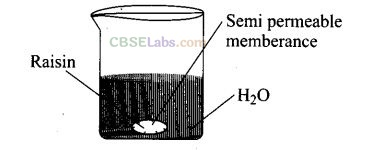Applications of the phenomenon

• Movement of water from soil into plant roots and subsequently into upper portion of the plant is partly due to osmosis.
• Preservation of meat against bacterial action by adding salt.
• Preservation of fruits against bacterial action by adding sugar. Bacterium in canned fruit loses water through the process of osmosis, shrivels and dies.
• Reverse osmosis is used for desalination of water.

Question 60. Discuss biological and industrial importance of osmosis.
Solution: Some essential importance of osmosis are given below:

• In animals, circulation of water to all parts of body takes place due to osmosis.
• Plant roots absorb water from soil due to osmosis. Concentration of cell sap inside the root hair cells is higher than that of water present in the soil. Water enters the root cells due to endosmosis.
• Water absorbed by plant roots is circulated in the entire plant body and reaches to the top of a tall tree due to osmosis.
• Osmosis helps in plant growth and germination of seeds.
• Red blood cells burst when placed in water; it is due to endosmosis.
• Various functions of plants are controlled by osmosis, e.g., stretching of leaves and flowers, opening and closing of flowers.
• Use of salt and sugar in‘pickles and jams acts as preservatives. It prevents growth of bacteria and fungi by osmosis.
• Dead bodies swell under water due to endosmosis.
• When dried fruits and vegetables are placed in water, they slowly swell and return to the original form. It is again due to endosmosis of water into the fruits and vegetables.
• Edema: Due to excess intake of salt by a person, the tissues become puffy, it is called edema. It is due to retention of water in the tissue owing to osmosis.

Question 61. How can you remove the hard calcium carbonate layer of the egg without damaging its semipermeable membrane? Can this egg be inserted into a bottle with a narrow neck without distorting its shape? Explain the process involved.
Solution: This can be achieved as under:
(i) Place the egg in a mineral acid solution for about 2 hours. The outershell of the egg dissolves. Remove any portion of it is left with your fingers.
(ii) Place the egg in a saturated solution (hypertonic) of sodium chloride for about 3 hours. Size of the egg is reduced as the egg shrivels due to osmosis.
(iii) Insert the egg in a bottle with a narrow neck. Add water to the bottle. Water will act as hypotonic solution, Egg regains shape due to osmosis. This is shown diagrammatically as under: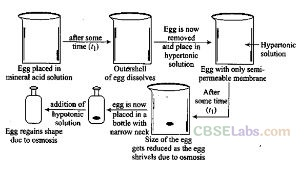Question 62. Why is the molar mass determined by measuring a colligative property in case of some solutes abnormal? Discuss it with the help of van’t Hoff factor.
Solution: The compounds which dissociate or associate in the solvent show abnormal molecular masses.
(i) Association: Compounds like benzoic acid or ethanoic acid dimerise in benzene due to hydrogen bonding as a result of which the number of particles in the solution decreases. Since colligative properties depend upon number of particles, such solutes show lower colligative property.
(ii) Dissociation: Electrolytes like NaCl, KC1, etc. dissociate into ions which result in increased number of particles, hence higher value of colligative property.
To account for association or dissociation van’t Hoff introduced a factor T known as van’t Hoff factor. It is defined as Expected molar mass Abnormal molar mass _ Observed colligative property Calculated colligative property
Total number of moles of particles _ after association/dissociation Total number of moles of particles before association/dissociation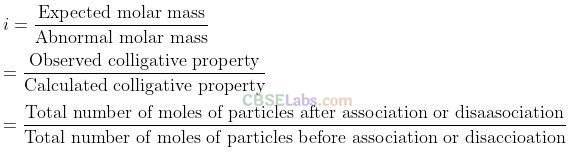We hope the NCERT Exemplar Class 12 Chemistry Chapter 2 Solution help you. If you have any query regarding NCERT Exemplar Class 12 Chemistry Chapter 2 Solution, drop a comment below and we will get back to you at the earliest.